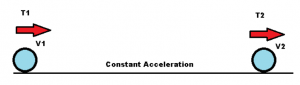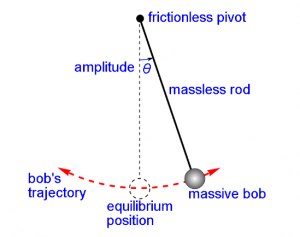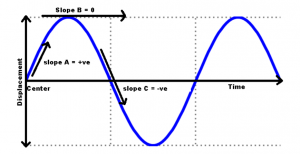# Displacement as a function of time and Periodic function

To understand this idea of displacement as a function of time, we will have to derive an expression for displacement, assume a body traveling at an initial velocity of $$v_1$$ at the time $$t_1$$ and then the body accelerates at a constant acceleration of ‘$$a$$’ for some time and a final velocity of $$v_2$$ at the time $$t_2$$, keeping these things in assumption let’s derive the following.Let’s write displacement as,

$$d$$ = $$V_{average}*\Delta t$$

Where $$\Delta t$$  is the change in time, assuming that the object is under constant acceleration.

$$d$$ = $$(\frac{V_1 + V_2}{2})* \Delta t$$

Where $$V_2$$ and $$V_1$$ are final and initial velocities respectively, let’s rewrite final velocity in terms of initial velocity for the sake of simplicity.

$$d$$=$$(\frac{V_1 + (V_1~+~a*\Delta t)}{2})* \Delta t$$

Where $$a$$  is the constant acceleration the body is moving at, now if we rewrite the above as,

$$d$$ = $$(\frac{2*V_1}{2} + \frac{a*\Delta t}{2})*\Delta t$$

The above expression is one of the most fundamental expressions in kinematics, it is also sometimes given as

$$d$$ = $$V_{i} t~+~\frac{1}{2}at^2$$

Where $$V_i$$ is the initial velocity and $$t$$ is actually the change in time, all the quantities in this derivation like Velocity, displacement and acceleration are vector quantities.

If we have a look at our final expression, it is very clear that change in displacement depends upon time.

## Example of an oscillating pendulumNow if we draw a displacement-time graph of this oscillating pendulum bob, we will get something like this,The graph of the motion of the bob shows us how the displacement varies with respect to the time when bob reaches the highest point, the potential energy is maximum and the kinetic energy is minimum as the velocity is equal to zero, we can easily deduce that the velocity is equal to zero, by looking at the slope of the displacement-time graph at specific times.

Slope A is positive in the graph stating that the velocity of the body is also positive, or in the forward direction.

Slope B is equal to zero meaning that the velocity of the body is zero, meaning there is no motion at this point.

Slope C is negative in the graph stating that the velocity of the body is also negative, or in the reverse direction.

The magnitude of velocity is always positive, it is the direction that decides if the velocity is positive or negative.

The displacement of the bob repeats after a certain amount of time, it is a periodic function hence the displacement at any given time in the future can be predicted if we know the time and the time period of the pendulum, hence we can say that displacement is a function of time.

To learn concepts in a simplified way join us here at BYJU’S.

Test your knowledge on Displacement periodic function